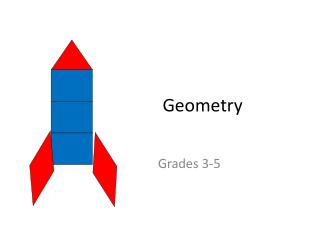DownloadDownload PresentationGeometry

# Geometry

Download Presentation## Geometry

- - - - - - - - - - - - - - - - - - - - - - - - - - - E N D - - - - - - - - - - - - - - - - - - - - - - - - - - -
##### Presentation Transcript

2. Goals: • Build an understanding of the mathematical concepts within Geometry, Measurement, and NBT Domains • Analyze and describe how concepts of geometry and geometric measurement progress through the grades • Engage in math tasks that make connections among domains • Explore Geometry Tasks and Resources to Take Back to Your Classroom

3. CCSS Goals for Elementary Geometry • Geometric shapes, their components (e.g. sides, angles, faces), their properties, and their categorization based on those properties. • Composing and decomposing geometric shapes. • Spatial relations and spatial structuring.

4. Progression of Geometry and Geometric Measurement • Domain: Geometry (G) and Measurement & Data (MD – measurement progression) • Read and make note of the the main concepts of grades K-5 • Describe how K-5Geometry Standards connect to the K-5 MD and NBT Standards • Identify how the concept changes and increases in rigor and understanding for the student • What benefits might be gained from understanding the progression?

5. Components, Properties, and Categorization of Geometric Shapes Concepts included build on our K-2 work from the morning. The activities are similar with increased sophistication. Examples: • Venn diagrams • Guess my rule • Sorting • Flow chart

6. Composing and Decomposing Geometric Shapes Assemble your tangram pieces into one large square.

7. Connecting the measurement and number • What if the area of the small square piece is ONE SQUARE UNIT? What is the area of each of the other pieces? What is the area of the entire large square? • What if the area of the large square is ONE SQUARE UNIT? What is the area of each of the seven smaller pieces?

8. Assume the perimeter of the small square is FOUR UNITS. What is the perimeter of each of the seven pieces, and what is the perimeter of the large square?

9. Jerry says, “I did a big triangle instead of a big square. Even if the small square is still ONE SQUARE UNIT, my answers for the area and perimeter of the big triangle will be different than we found for the big square.” Tom disagrees, “If the small square is still ONE SQUARE UNIT, my big rectangle will have the same area and perimeter as the big square because we are using all the same pieces even if they are making a different shape.”

10. Spatial Relations and Structuring • Mental operation of constructing an organization or form for an object or set of objects in space • Precedes meaningful mathematical use of multiplication, area, volume, number properties, and the coordinate plane

11. Quick Rectangles • Build the rectangle you see flashed on the screen with square tiles. • Draw the rectangle you see flashed on the screen. What mathematical issues are raised when you have to draw instead of build?

12. Commutative Propertya x b = b x a • How many rectangles can you make with an area of 12 square tiles?

13. How many cards are needed? A club wants to create a card section at the next football game by placing a card on each seat in a certain section. They are planning to use a section of seats that has 5 rows, and then plan to put 3 yellow cards, then 4 blue cards, and then 5 green cards down each row. How many cards altogether will be needed so that each seat in this section has a card on it? Write number sentences to show how you found your answer. From Benson, Wall, & Malm (2013)

14. How many cards are needed? From Benson, Wall, & Malm (2013)

15. Distributive Property 5 x 12 = 5 x (3 + 4 + 5) = (5 x 3) + (5 x 4) + (5 x 5) A x (B + C) = (A x B) + (A x C)

16. Multiplying Larger Numbers • How can you use the base ten blocks to show 12 x 14 using the least number of pieces possible?

17. Connecting to Written Algorithms Partial Products Algorithm 2 10 14 X 12 8 20 40 + 100 40 4 8 20 100 10

18. 3-D Spatial Structuring Build a Building • What patterns do you see as you continue to add stories to each building? • How could you use these patterns to figure out the number of rooms even without building?

19. 3-D Spatial Structuring • Describe the rectangular prism on your table. • What are all the different ways to find the number of cubes it took to build it? • Look at the net for this prism. How does it relate to the 3-D shape?

20. How many other rectangular prisms can be made with 24 cubes? • Build • Draw the nets, cut out and make them • Use the cm paper to make the biggest rectangular prism possible in terms of volume (you must draw the net from the paper).

21. Resources • Check out LAUNCH Blog for today’s power point and other websites and resources for teaching geometry.